# Python 语法之数据类型

• 2022 年 9 月 20 日
四川
• 本文字数：5623 字

阅读完需：约 18 分钟

name,age,weight = '女娲',18,49.99print(name)print(age)print(weight)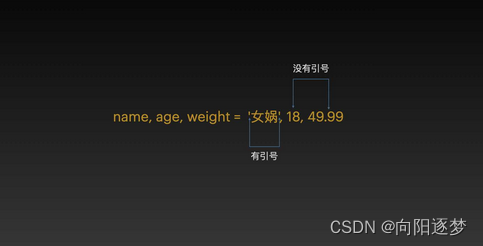## 1.数字类型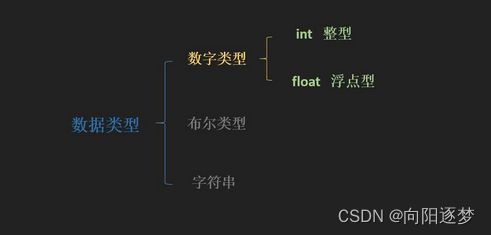（1）int

• 相传在上古时期，老百姓无法分辨粮食和草药，只能依靠打猎维持生存，即使生病也没办法医治，神农氏因为想改变这个局面，亲自上山采药，亲口尝各类草药，辨别药性。神农氏踏遍了山野，尝了七七四十九天，发现了麦、稻、谷子、高粱能充饥，并且尝出了 365 种草药，还编写了《神农本草》。

• 在这个故事中，我们看到了 2 个整数，七七四十九天和 365 种草药。

day = 49number = 365print(day)print(number)

• 根据变量命名规则，我们将整数 49 赋值给变量 day，整数 365 赋值给变量 number。 整数为正数或负数，没有小数，长度不限。这些都是整数哟！

#光速，每秒30万公里speed_of_light = 300000   #地球质量，5.97×10²⁴kgmass_of_earth = 5970000000000000000000000#地球最深峡谷的海拔，10994m，因在地下，我们这里用负数表示altitude_of_mariana_trench = -10994print(speed_of_light)print(mass_of_earth)print(altitude_of_mariana_trench)

• 我们可以通过下划线_将有含义的英文连接起来，让我们的代码显得规范。（2）float

• 浮点数是包含小数的正数或负数，就是我们人类世界中的小数。

• 比如大家非常熟悉的 π：pi = 3.1415926，π 是特殊字符，不能用作变量名，因此我们这里用谐音 pi 来代替。

pi = 3.1415926negative_pi = -3.1415926print(pi)print(type(negative_pi))

（3）运算符

• 我们大家都知道数字涉及到加减乘除、比大小等各种运算，Python 中也有这些运算哦，这就是 Python 的运算符，Python 中运算符包括算术运算符、赋值运算符、比较运算符和逻辑运算符，我们逐一来介绍。

•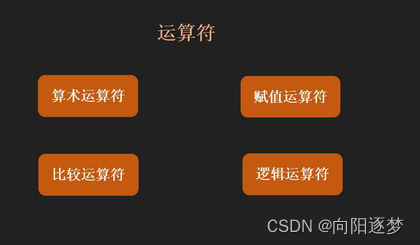• （3.1）算数运算符

• 我们再来回顾下神农尝百草的故事，神农氏踏遍了山野，尝了七七四十九天，发现了麦、稻、谷子、高粱能充饥。

• 这里面的七七十九天，就可以用算术运算符来表示哦，Python 中用*来表示两数相乘，具体语句是：

day1 = 7day2 = 7day = day1 * day2print(day)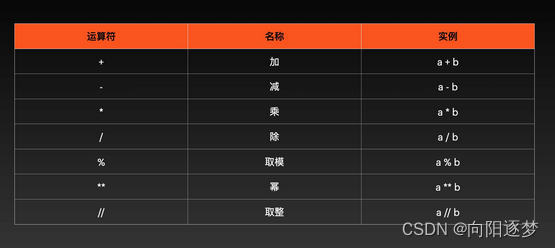（3.1.1）+（加）运算符

a = 1b = 1c = 3.14d = 2.71print(a + b)print(c + d)

（3.1.2）-（减）运算符

a = 1b = 1c = 3.14d = 2.71print(a - b)print(c - d)

（3.1.3）*（乘）运算符

a = 2b = 1c = 3.14d = 2.71print(a * b)print(c * d)

（3.1.4）/（除）运算符

a = 2b = 1c = 3.14d = 2.71print(a / b)print(c / d)

（3.1.5）%（取模）运算符

a = 10b = 3print(a % b)

（3.1.6） ** （幂）运算符

a = 10b = 3print(a ** b)

（3.1.7）//（取整）运算符

a = 10b = 3print(a // b)

（3.2）赋值运算符下面，我们一起来看下赋值运算符，在变量的赋值中，我们提到了 = 的含义是赋值，在 Python 中赋值运算符 = 可以和算术运算符进行结合。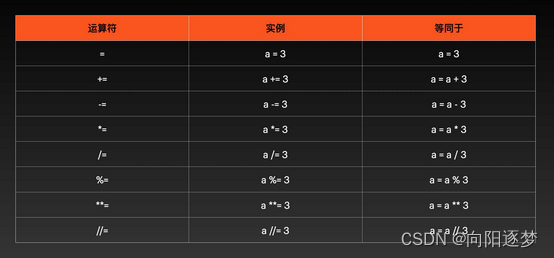（3.2.1）=运算符= 运算符和变量的赋值是一样的。

a = 3  # =运算符print(a) number = 365number += 30 # +=运算符print(number)a = 3a -= 3 # -=运算符print(a)a = 3a *= 3 # *=运算符print(a)a = 3a /= 3 # /=运算符print(a)a = 10a %= 3 # %=运算符print(a)a = 10a **= 3 # **=运算符print(a)a = 10a //= 3 # //=运算符print(a)

（3.3）比较运算符

• 在神农品尝的 365 种草药中，假设有 50 种可以作为粮食食用，有 200 种是对人类疾病有益的药材，那么到底是药材的数量多还是粮食的数量多呢，这就涉及到比较啦，在人类的认知中，这种比较非常简单：200 >50，那么在 Python 的世界中，这种比较是如何表达的呢，比较运算符就可以解决这个问题！

food_number = 50drug_number = 200print(food_number < drug_number)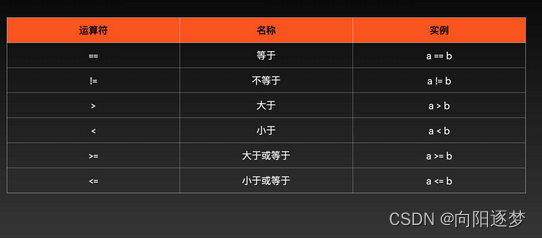==（等于）比较运算符 和 = 赋值运算符的区别：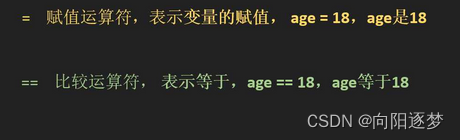• 在我们平时的认知中，等于就是用等号 = 来表达，但是在计算机中，等于要用 2 个等号来表达，单个等号 = 的含义是赋值哦，这一点，大家要熟记哈！

a = 1b = 2print(a == b)

（3.4）逻辑运算符

• 神农告诉人们，麦、稻、谷子、高粱等 50 种植物可以作为粮食充饥，蒲公英、黄芪、三七等 200 种植物可以作为药材，现在我们想判断下粮食的数量小于 60 并且药材的品种大于 150，是否是真实的，该如何判断呢？在 Python 中是通过逻辑运算符 and 来实现的，我们来看下方语句：

food_number = 50drug_number = 200print(food_number < 60 and drug_number > 150)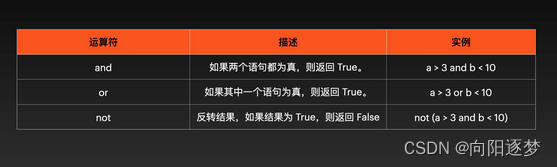and

a = 4b = 8print(a > 3 and b < 10)print(a > 3 and b < 6)

• 我们看到当 a 赋值为 4，a > 3 为真，b 赋值为 8，b < 10 为真，b < 6 为假，逻辑运算符 and 当 a 和 b 都为真的时候，才会返回 True，因此第一个 print() 返回值为 True，第二个 print() 返回值为 False。

## 2.布尔型

• 我们在进行逻辑运算和比较运算的时候，得到的结果为 True 或 False。在 Python 中，用 True、False 表示布尔值。布尔值可以用 and、or 和 not 运算。2.1 and 运算布尔值的 and 运算就是真真为真，当 and 连接的两个布尔型都是 True 的时候，为 True ，一真一假和两假都为假。

print(True and True)print(True and False)print(False and False)

2.2 or 运算布尔值的 or 运算就是假假为假，当 and 连接的两个布尔型都是 False 的时候，为 False ，一真一假和两真都为真。

print(True or True)print(True or False)print(False or False)

2.3 not 运算布尔值的 not 运算则表示非，非真就是假，非假就是真！

print(not True)print(not False)

## 3.字符串类型

print('hello world!')print("hello world!")

print('''Beautiful is better than ugly.Explicit is better than implicit.Simple is better than complex.Complex is better than complicated.Flat is better than nested.Sparse is better than dense.''')

print("""Beautiful is better than ugly.Explicit is better than implicit.Simple is better than complex.Complex is better than complicated.Flat is better than nested.Sparse is better than dense.""")

say_hi = "hello world!"print(say_hi)

3.1 索引中国古人一直有观天象的习俗，通过观天象，来获得对季节、气候、甚至是国家命运的认知。现代，通过科学家们的努力，我们知道了浩瀚宇宙的深邃，知道了八大行星的存在，海王星(neptune)，八大行星之一，假设我们在 Python 的世界中，可以重见神农，让我们用 Python 的语言为神农介绍下海王星的字母构成吧！这就要运用我们下方为大家介绍的内容：索引。在 Python 中，字符串中的字符可以通过索引来提取。可以从前往后索引，也可以从后往前索引。索引语法是：变量[下标]，这里的下标是由数字表示，代表所要索引的字符在变量中的位置。当从前往后索引时，下标从 0 开始。当从后往前索引时，下标从 -1 开始，如下图所示！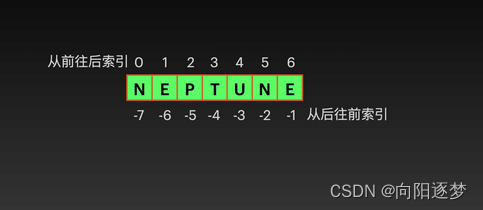star = "NEPTUNE"print(star)print(star[-1])print(star)print(star[-7])

star = "NEPTUNE"# 获取 NEPprint(star[0:3])# 获取 NEPTUNEprint(star[:])# 获取 NEprint(star[-7:-5])# 获取 EPTUNEprint(star[1:])# 获取 NEPTprint(star[:4])

3.3 字符串长度神农：“现代社会真神奇啊，不仅能认识海王星，还能读取海王星的另一种语言表达，可是我还有一个疑问，海王星的英文是几个字母构成的呢”？我们：“这个问题简单，Python 中 len() 可以获取字符串的长度哦”

star = "NEPTUNE"print(len(star))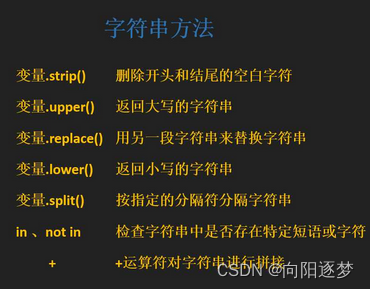strip() 删除开头和结尾的空白字符神农：“现代的信息技术太精彩了！”我们：“这都不算什么哟，还有更精彩的，同样的 NEPTUNE（海王星），我们也可以做出一些变换的哦”神农：“哦？快快，教教我是怎么做的”有些时候，我们会在代码的编写中做一些空格的操作，这些空格在 Python 中就是空白字符，我们可以使用 strip()来删除字符串开头和结尾的空白字符，使用方法就是变量名.strip()，我们来看下方语句：

star = " NEPTUNE "print(star)print(len(star))print(star.strip())print(len(star.strip()))

print("You're uinique, nothing can replace you.")

（2）由单引号 ' 括起来的任意文本包含双引号 "

print('The man who has made up his mind to win will never say "impossible".')

print('You're uinique, nothing can replace you.')print("The man who has made up his mind to win will never say "impossible"")

## 4.获取数据类型与数据类型转换

#变量weight1，赋值为整数50weight1= 50#变量weight2，赋值为字符串50weight2 = '50'#变量weight3，赋值为浮点数50.00weight3= 50.00#打印变量weight1的数据类型print(type(weight1))#打印变量weight2的数据类型print(type(weight2))#打印变量weight3的数据类型print(type(weight3))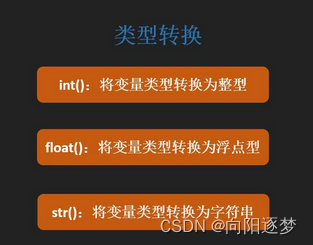weight1= 50weight3= 50.00weight4= int(weight3)weight5=float(weight1)print(type(weight1))print(type(weight3))print(type(weight4))print(type(weight5))

num1 = 3num2 = 50num3 = 150print('神农每天品尝 '+str(num1)+' 种草药，品尝了 '+str(num2)+' 天，一共品尝了 '+str(num3) + ' 种草药')### 向阳逐梦

InfoQ签约作者、阿里云“乘风者计划”签约博主

## 评论